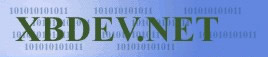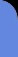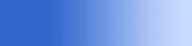Saturday July 4, 2020
 home | about | contact | DonationsOpenXDK The Free, Legal, Open Source XBox Development Kit...

Basic Animation - Bouncing Ball

Well we get 50fps, so using this we can do some simple animation...nothing to advanced yet...we'll just have a ball bounce around the screen.

 Project Source Code - Bouncy Ball // Few lines of code that demonstrate the basic graphics setup so you can work // on doing some simple displays etc   #include      void xbvDrawCircle( unsigned int *pdwFramebuffer, int nSizeStrideInDwords, DWORD dwPixel, int xC, int yC, int r ) {       int x=0, y=r, u=1, v=2*r-1, e=0;         while( x < y ){               pdwFramebuffer[(xC+x)+((yC+y)* nSizeStrideInDwords)]=dwPixel;             pdwFramebuffer[(xC+y)+((yC-x)* nSizeStrideInDwords)]=dwPixel;             pdwFramebuffer[(xC-x)+((yC-y)* nSizeStrideInDwords)]=dwPixel;             pdwFramebuffer[(xC-y)+((yC+x)* nSizeStrideInDwords)]=dwPixel;             x++; e += u; u+= 2;             if( v < 2 * e ) { y--; e -= v; v -= 2; }             if( x > y ) break;             pdwFramebuffer[(xC+y)+((yC+x)* nSizeStrideInDwords)]=dwPixel;             pdwFramebuffer[(xC+x)+((yC-y)* nSizeStrideInDwords)]=dwPixel;             pdwFramebuffer[(xC-y)+((yC-x)* nSizeStrideInDwords)]=dwPixel;             pdwFramebuffer[(xC-x)+((yC+y)* nSizeStrideInDwords)]=dwPixel;       }   }// end of xbvDrawCircle(..)   volatile DWORD time; void sleep( DWORD ms ) {     DWORD Target = KeTickCount+ms;         while(1){             time = KeTickCount;             if( time > Target ) break;       } }// end of sleep(..)     // Program Entry Point void XBoxStartup() {         //let's init the screen       vga_init_mode(MODE_640x480x32);         // Some possible modes all defined in                                               // xvga.h                                               // MODE_320x240x32                                               // MODE_640x240x32                                               // MODE_640x480x32 etc...plus many more           float xpos = 640/2;       float ypos = 640/2;         float xdir = 0.5f;       float ydir = 1.0f;         while(1)       {             // A list of all these functions can be found at the top of xvga.h             // Comment this line out to watch the ball go crazy! - why is that..well             // we have to wait a bit for it to finish its blitz to the screen and it slows             // us down...might be better to do multiple back buffering?             vga_vsync();                                    // Wait for Vertical Blank         vga_flip();                                         // Flip             // Comment this line out to see the effects of the ball going around the screen,             // sort of a trail of itself, you can also see how far it moves with each movement             vga_clear();                                    // Clear screen               ScreenInfo s = vga_get_screen_info();               unsigned int* pix = (unsigned int*)s.ScreenAddress;                         // Draw new circle in a new place             xbvDrawCircle( pix, 640, 0xffffff00, (int)xpos, (int)ypos, 10 );               xpos += xdir;             ypos += ydir;               int size = 10; // diameter of our little circle             if( (xpos <= (0+size)) || (xpos >= (640-size)) ) xdir = -xdir;             if( (ypos <= (0+size)) || (ypos >= (480-size)) ) ydir = -ydir;                   //sleep(50);         }// End while loop }//End

 Visitor: 9534626  { 209.237.238.175 } Copyright (c) 2002-2020 xbdev.net - All rights reserved. Designated tutorial and software are the property of their respective owners.